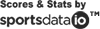2022 Positional Spending

spending by positional category, including pitchers, catchers, infielders, outfielders, & designated hitters.

Allocation: Infield Roster

Player Option
Club Option
Mutual Option
Qual. Offer
Estimate
Rank Player Position Dollars % of
Totals \$0 0.00%
Average \$nan nan%
Totals \$0 0.00%
Average \$nan nan%
Totals \$0 0.00%
Average \$nan nan%
Totals \$0 0.00%
Average \$nan nan%
Totals \$0 0.00%
Average \$nan nan%
Totals \$0 0.00%
Average \$nan nan%
Totals \$0 0.00%
Average \$nan nan%
Totals \$0 0.00%
Average \$nan nan%
Totals \$0 0.00%
Average \$nan nan%
Totals \$0 0.00%
Average \$nan nan%
Totals \$0 0.00%
Average \$nan nan%
Totals \$0 0.00%
Average \$nan nan%
Totals \$0 0.00%
Average \$nan nan%
Totals \$0 0.00%
Average \$nan nan%
Totals \$0 0.00%
Average \$nan nan%
Totals \$0 0.00%
Average \$nan nan%
Totals \$0 0.00%
Average \$nan nan%
Totals \$0 0.00%
Average \$nan nan%
Totals \$0 0.00%
Average \$nan nan%
Totals \$0 0.00%
Average \$nan nan%
Totals \$0 0.00%
Average \$nan nan%
Totals \$0 0.00%
Average \$nan nan%
Totals \$0 0.00%
Average \$nan nan%
Totals \$0 0.00%
Average \$nan nan%
Totals \$0 0.00%
Average \$nan nan%
Totals \$0 0.00%
Average \$nan nan%
Totals \$0 0.00%
Average \$nan nan%
Totals \$0 0.00%
Average \$nan nan%
Totals \$0 0.00%
Average \$nan nan%
Totals \$0 0.00%
Average \$nan nan%